# UNDERSTANDING COMPOUNDING & COMPOUNDING EFFECTS

## Compounding is relevant for almost every financial evaluation for periods of longer than a year.  A sound understanding of compounding and its effects is an essential building block of your financial awareness.

1.  Perform compounding calculations period by period.

3.  Apply short cut formulae with full understanding.

## 1.  Compounding period by period

Compound interest is based on interest on interest, as well as interest on the original amount borrowed or invested.

Let’s say we invest \$100m for two years, at a compound interest rate of 20% per year.

Year 1 interest calculation

Our interest for the first year is:

\$100m x 20% = \$20m

The total value of our investment has grown to:

\$100m + \$20m = \$120m

For the first year, compounding hasn’t had any effect yet.  There was no accumulated interest brought forward, on which to calculate any interest on interest.

Year 2 interest calculations

In the second year, compounding starts to make a difference to our results.

Interest for the second year is:

(1)  A further 20% on our original amount:

\$100m x 20% = \$20m

(2)  Plus compound interest of 20%, on our \$20m interest brought forward from Year 1:

\$20m x 20% = \$4m

Our total interest for Year 2:

\$20m + \$4m = \$24m

By the end of Year 2, the total value of our investment has grown to:

\$120m + \$24m = \$144m

Compounding has resulted in an additional \$4m of interest so far (= \$100m x 20% x 20%).

Interest on our original amount accumulates  steadily at \$20m per year

## 01 cumulative interest on original amount.JPGYear by year and cumulative totals – Years 1 to 4

## 02 year by year and cumulative totals years one to four.JPG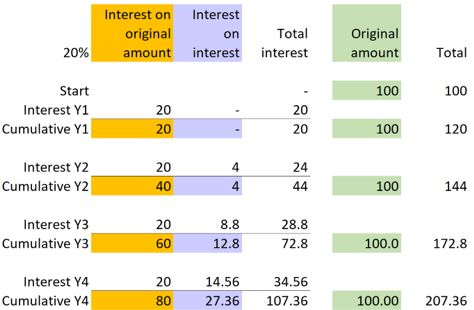Year 3 calculations

Now let’s check the calculations in our table for Year 3.

Interest for the third year is:

(1)  A further 20% on our original amount:

\$100m x 20%  = \$20m

(2)  Compound interest of 20% on our cumulative interest of \$44m at the end of Year 2:

\$44m x 20% = \$8.8m

Total interest for Year 3:

\$20m + \$8.8m = \$28.8m

By the end of Year 3, the total value of our investment has grown to:

\$144m + \$28.8m = \$172.8m

Interest on interest accumulates by ever-increasing annual amounts

## 03 cumulative interest on interest v years.JPG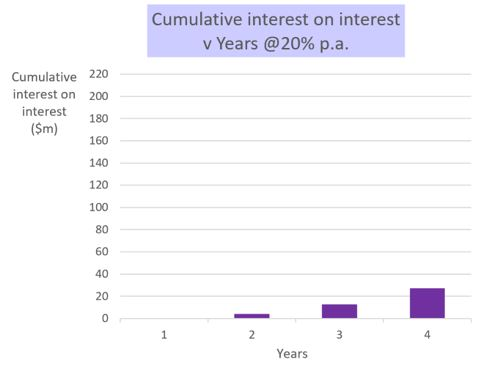Interest on interest is why the future total grows by an ever-increasing value per year

## 04 total amount v years.JPG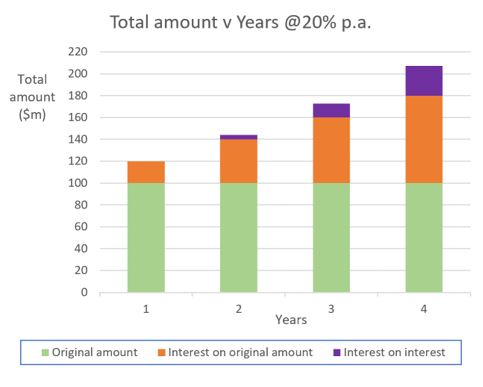Interest on interest grows as a proportion of the total – for example Years 5 to 20

## 05 total amount v years.JPG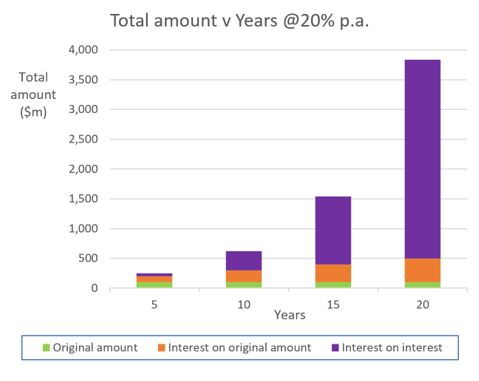Exactly the same relationships apply whether we are working with interest, other forms of return, or any other kind of growth.

Please confirm your understanding by recalculating the interest amounts and our future total for Year 4.  (The answer is at the end.)

The lower the rates, the smaller the compounding effects – for example 2%

## 06 total amount v years.JPG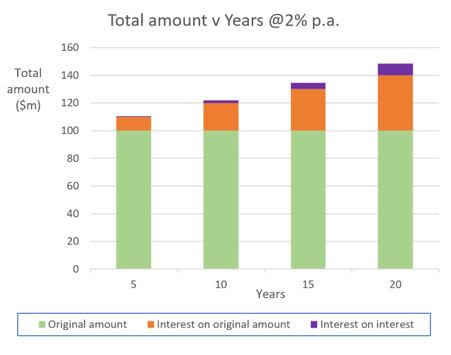At a rate of 2% per year, illustrated above, the interest on interest amounts - and proportions of the total - are much smaller than they were at the rate of 20% per year.

At just 0.2% per year, compounding effects are negligible, even after 20 years

## 07 total amount v years.JPG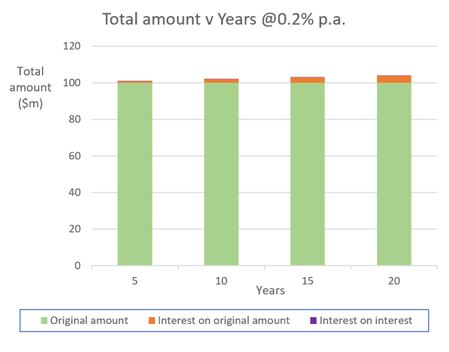## 2.  Summarising what we’ve learned so far – sense-checks

• When rates of interest, growth or return are positive, compounding results in greater total values.
• The greater the rates of interest, growth or return, the larger the compounding effects.
• The more periods that compounding is applied for, the greater the compounding effects.
• When lower rates are applied for smaller numbers of periods, compounding effects are small.
• Compounding effects are greater when rates are higher, or the number of periods is more, or both.

## 3.  Is there a short-cut formula for compounding?

There is.

To work out the total future amount by formula, the Future Value (FV):

FV = PV  x  (1 + r)^n

Where:

PV = Present value, the value today

r = interest rate per period

n = number of periods

Example – 3 years at 20% per year

PV = \$100m

r = 20% = 0.20

n = 3

FV = PV  x  (1 + r)^n

= 100  x  (1 + 0.20)^3

= 100   x  1.728

= \$172.8m, as before

Are there short cut formulae for the interest amounts?

Yes indeed, there are three of them.

(1) Total cumulative interest = FV - PV

Where:

FV = Future value, the future amount

PV = Present value, the value today

Using the same example – 3 years at 20% per year

FV = \$172.8m, calculated above

PV = \$100m

Total cumulative interest = FV - PV

= 172.8 – 100

= \$72.8m, as in our table above

(2) Cumulative interest on original amount = PV  x  r  x  n

Where:

PV = Present value, the value today

r = interest rate per period

n = number of periods

Continuing the same example – 3 years at 20% per year

PV = \$100m

r = 20% = 0.20

n = 3

Cumulative interest on original amount = PV  x  r  x  n

= 100  x  0.20  x  3

= \$60m, as in our table above

(3) Cumulative interest on interest = Total interest – interest on original amount

Completing the same example – 3 years at 20% per year

Cumulative interest on interest = Total interest – interest on original amount

= 72.8 – 60

= \$12.8m, as in the table

Confirming the calculations in our table for Year 4.

Interest for the fourth year is:

(1)  A further 20% on the original amount:

\$100m x 20% = \$20m

(2)  Compound interest of 20% on the \$72.8m cumulative interest at the end of Year 3:

\$72.8m x 20% = \$14.56m

Total interest for Year 3:

\$20m + \$14.56m = \$34.56m

The total value of the investment has grown to:

\$172.8m + \$34.56m

= \$207.36m, as in the table.

Many congratulations!

You have mastered a very important and challenging financial concept.

___________________

Author: Doug Williamson, FCT